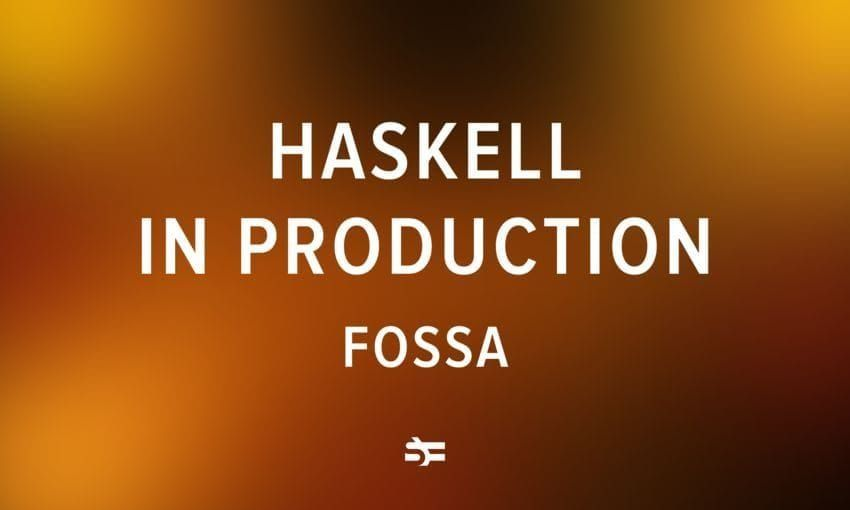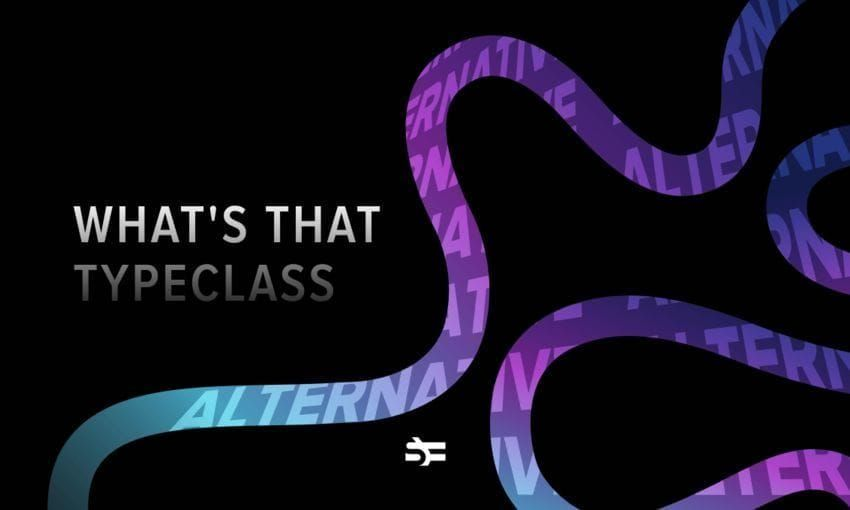# What's That Typeclass: Monoid

In programming, there’s a pattern that occurs very frequently – putting together two things of the same type to get another thing of that type.

a -> a -> a


Given its frequency, it would be nice to have some useful abstractions for it.

And in Haskell, we do. There’s a typeclass called Monoid that abstracts over the notion of “smashing things together”. It draws inspiration from a mathematical structure with the same name.

By the end of the article, you will know:

• what is a monoid;
• what is the Monoid typeclass in Haskell;
• how to use predefined monoid instances from Data.Monoid;
• how to define your own instances of Monoid;
• why are monoids useful.

Recommended previous knowledge: algebraic data types and typeclasses.

## Building up intuition

With math terms like monoid, it’s always better to look at examples before reading the definition.

Therefore, let’s look at three examples of monoid-like behaviors in Haskell.

First off, lists with the ++ (concatenation) operator.

Prelude> [1,2] ++ [3, 4] -- You can concatenate lists.
[1,2,3,4]
Prelude> [1,2] ++ [] -- Concatenating an empty list to a list doesn't change the result.
[1,2]
Prelude> [] ++ [1,2]
[1,2]
Prelude> [1,2] ++ ([3,4] ++ [5,6]) -- It doesn't matter in which order you concatenate the lists, you get the same result either way.
[1,2,3,4,5,6]
Prelude> ([1,2] ++ [3,4]) ++ [5,6]
[1,2,3,4,5,6]


After that, numbers with the + operator.

Prelude> 1 + 2 -- You can sum two natural numbers together.
3
Prelude> 1 + 0 -- Adding a 0 doesn't change the sum.
1
Prelude> 0 + 1
1
Prelude> (1 + 2) + 3 -- It doesn't matter in which order you sum the numbers, you get the same result either way.
6
Prelude> 1 + (2 + 3)
6


(Alternatively, we could have also done numbers with the * operator. In that case, the element that doesn’t change the product would be 1.)

And, finally, booleans with the && operator (which stands for and).

Prelude> True && False -- You can join two booleans via &&.
False
Prelude> a = False
Prelude> a && True -- Adding && True doesn't impact the resulting boolean.
False
Prelude> True && a
False
Prelude> (True && True) && False -- It doesn't matter in which order you resolve the &&s, you get the same result either way.
False
Prelude> True && (True && False)
False


(Alternatively, we could have done booleans with the or operator: ||. In that case, the element that wouldn’t change the result would be False.)

As you can see, these three things act similarly. They follow the same laws.

Now, let’s look at what exactly these laws are.

## What is a monoid?

In mathematics, a monoid is a structure that consists of a set of elements (such as numbers or booleans) and a binary operation on that set (such as + or &&).

In addition, a monoid satisfies the following properties:

• There is an identity element that “doesn’t do anything”. In more formal terms, if you take any element x from the monoid’s element set and use the binary operation of the monoid with that element and the identity element, you will get the same element – x – back. E.g., $1 + 0 = 1$, $2 + 0 = 2$, etc.
• Associativity. This property guarantees that rearranging the parentheses in the equation won’t change the result of the equation. E.g., $(1 + 2) + 3 = 1 + (2 + 3)$.

### Monoid typeclass in Haskell

How does a monoid look in Haskell? :info detective is on the case. 🕵️‍♂️

Prelude> :info Monoid
type Monoid :: * -> Constraint
class Semigroup a => Monoid a where
mempty :: a
mappend :: a -> a -> a
mconcat :: [a] -> a


As we can see, Monoid in Haskell is a typeclass that has three methods: mempty, mappend, and mconcat.

• mempty is a value that doesn’t impact the result when used together with the binary operation. In other words, it’s the identity element of the monoid.
• mappend (or <>) is a function that puts two monoids together. In other words, it’s the binary operation of the monoid.
• mconcat is a function that reduces a list of monoids into one value. By default, it’s foldr mappend mempty. That’s fine for most data types, and it’s not necessary to define mconcat to define an instance. But you might sometimes want to define your own implementation of the function when the default implementation is not optimal.
Note on mappend and <>.

While the typeclass doesn’t define a function called <>, it’s defined by Semigroup , the superclass of Monoid that I’ll cover later in the article. For all intents and purposes, these functions should be the same. In a future release of GHC, mappend will be removed, so you are advised to use <>.

## How to use predefined monoid instances

Let’s try to use these monoid methods with data types from our previous examples.

It works with lists.

Prelude> [1,2] <> [3,4]
[1,2,3,4]

Prelude> mempty :: [a]
[]

Prelude> [1,2] <> mempty
[1,2]


But if you try to do 1 <> 3, you will be greeted by an error in GHCi.

<interactive>:1:1: error:
• Ambiguous type variable 'a0' arising from a use of 'print'
...
🤔


This happens because there’s no single instance of Monoid for numbers.

You can have a sum monoid, a product monoid, and many more, depending on the binary operation you choose. GHC has no way of knowing which operation you want to use.

So we need to wrap our data types and make monoid instances according to the context those types will be used in. Data.Monoid defines such wrapper types for commonly used monoids like Sum, Product, etc.

newtype Sum a = Sum { getSum :: a }


Let’s see how they work.

Prelude> import Data.Monoid
Prelude Data.Monoid> Sum 1 <> Sum 3
Sum {getSum = 4}

Prelude Data.Monoid> Product 2 <> Product 5
Product {getProduct = 10}


Similarly, booleans have All and Any monoids defined for them.

Prelude Data.Monoid> All True <> All False
All {getAll = False}
Prelude Data.Monoid> Any True <> Any False
Any {getAny = True}


You can use mconcat to sum a list of these monoids.

result = mconcat [Sum 4, Sum 6, Sum 8, mempty]


And to get an unwrapped result, you can unwrap them via their record names, conveniently called getX.

Prelude Data.Monoid> getSum result
18


## How to create a monoid instance in Haskell

Let’s try creating our own monoid instance in Haskell.

First, we’ll create a custom datatype called Move that expresses instructions for a robot to move in a 2D field.

data Move = Move Int Int deriving (Show, Eq)


To create a monoid instance for a data type, you first need to create a Semigroup instance for it because Semigroup is a superclass of Monoid (since GHC 8.4).

Prelude> :info Monoid
type Monoid :: * -> Constraint
class Semigroup a => Monoid a where
...

Prelude> :info Semigroup
type Semigroup :: * -> Constraint
class Semigroup a where
(<>) :: a -> a -> a


But don’t worry, there’s nothing new here. Creating a Semigroup instance is just a roundabout way of defining mappend.

This is so because a semigroup is a monoid without an identity element ( mempty). It defines one method – <> – which is the same as mappend, the binary operation for combining two values.

So, let’s define an instance of Semigroup. To append two moves, we will sum their respective x and y values.

instance Semigroup Move where
Move x1 y1 <> Move x2 y2 = Move (x1 + x2) (y1 + y2)


After that, we can define the Monoid instance. To define the instance, you only need to provide mempty because mappend will be the same as <>.

The mempty that makes sense in our case is to not move anywhere: Move 0 0.

instance Monoid Move where
mempty = Move 0 0


Note: while creating your monoid instances, you need to be careful to follow the monoid laws. Haskell (barring property-based tests) has no way to check that our monoid instance makes sense. For example, we could have defined mempty to be Move 0 (-1), which would lead to some odd behavior.

That’s all we need for Move to be a monoid! 🎉

Now, we can play around with it in GHCi.

*Main> Move 3 4 <> Move 8 9
Move 11 13
*Main> Move 3 4 <> mempty
Move 3 4


We can also use the mconcat method to fold a list of moves.

*Main> stay = mempty :: Move
*Main> moves = [Move 1 2, Move (-3) 5, Move (-6) 3, stay, Move 2 3]
*Main> mconcat moves
Move (-6) 13


## Why do you need monoids?

In general, monoids are not very advanced, interesting, or cool.

However, they are really useful and quite simple. <> is used for concatenating a lot of different types and could be considered a basic building block. It’s an operator that you will meet in every Haskell project.

If you’re searching for real-life use cases, the further learning section below has resources that cover the use of monoids for making a chat filter and calculating electoral vote distributions.

## Further learning

This has been a quick introduction to the Monoid typeclass in Haskell. Hopefully, now you know what monoid laws are, how to use monoids in Haskell, and how to define your own instances of the Monoid typeclass.

Here’s some other resources on the subject that you might find helpful:

## Exercises

1. Implement a wrapper type for exclusive OR (XOR). Create Semigroup and Monoid instances for it.

Solution
newtype Xor = Xor {getXor :: Bool} deriving (Show, Eq)

instance Semigroup Xor where
Xor bool1 <> Xor bool2 = Xor (bool1 /= bool2)

instance Monoid Xor where
mempty = Xor False


2. Implement a wrapper type for logical biconditional (XNOR). Create Semigroup and Monoid instances for it.

Solution
newtype Xnor = Xnor {getXnor :: Bool} deriving (Show, Eq)

instance Semigroup Xnor where
Xnor bool1 <> Xnor bool2 = Xnor (bool1 == bool2)

instance Monoid Xnor where
mempty = Xnor True


3. Do the instances created in 1. and 2. follow the monoid laws? Why?

Solution

Answer: There are two laws that monoids need to satisfy: the existence of an identity element and associativity.

It’s easy to determine that False and True are the identity elements of XOR and XNOR, respectively, by looking at the truth tables of the operations.

Proving associativity is a bit harder, but here’s an intuitive proof on Stack Exchange for XOR. The one for XNOR should be equivalent in structure.

4. As you might remember, a semigroup is a monoid without the identity element. Can you come up with an example of a structure that is a semigroup but is not a monoid?

Solution

One example is non-empty lists with the ++ operator. While a regular list can be empty, and the empty list thus satisfies the requirement of an identity element, non-empty lists have no such element.

Serokell is a company that offers services in Haskell programming and has vast expertise in developing bespoke software solutions. We are happy to resolve your business challenges, be it finance, biotech, edtech, blockchain, or another sector. Let’s connect for a conversation!More from Serokell